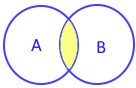# intersection() method in Python set

All set methods

Returns a new set with common elements only. Original set remains unchanged.

## intersection using & operatorAll Common elements in sets
Using ampersand ( & ) operator
``````A={1,2,3}
B={3,4,5}
print(A & B)``````
Output ( Note 3 is the only common element )
``{3}``

## Using type()

We can check the output by using type()
``````A={1,2,3}
B={3,4,5}
x=A & B
print(type(x))``````
Output
``<class 'set'>``

## Using intersection() method

``````A={'a','b','c'}
B={'a','y','z'}
print(A.intersection(B))``````
Output
``{'a'}``

## Using more than one sets

We can use any number of sets with intersection()
``````A={'a','b','c'}
B={'a','y','z'}
C={'a','k','l'}
print(A & B & C)``````
Output
``{'a'}``

## intersection() method

Using string ( iterable object ) with intersection() method
``````A={'a','b','c','x','y'}
B='Alex'
print(A.intersection(B))``````
Output
``{'x'}``
Using list with intersection() method.
``````A={'a','b','c'}
B=['a','x','y']
print(A.intersection(B))``````
Output
``{'a'}``
Below code will generate error.
``print(A & B)``
`` TypeError: unsupported operand type(s) for &: 'set' and 'list'``

## intersection_update()

In all above code by using intersection() we created a new set. By using intersection_update() method we can change the original set with common elements.

Subscribe to our YouTube Channel here

## Subscribe

* indicates required
Subscribe to plus2netplus2net.com